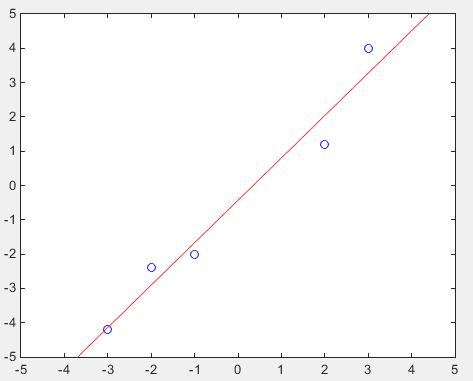Engineering Math - Quick Reference                                 Home : www.sharetechnote.com Least Square   'Least Square' is a special type of technique for data regression based on 'Sum of square of error between the given data and the estimated function(regression equation). Does this make sense to you ? Don't worry if this does not make sense to you. It doesn't make sense to me either :)   Let's take more practical (intuitive approach). Let's assume that somebody gave you the following 5 data points in the form of (x(n),y(n)) which can be plotted as shown below and he ask you to find a single straight line (a linear function) that can best represent (best fit) the whole data set. I assume that everybody would understand the linear function can be expressed as y = a x + b (this is a function from junior highschool math). You can change the location and the slope of the line (blue line in the following plot) by applying various different value for 'a' and 'b'. Now the question become 'How can you figure out the value for 'a' and 'b' to make the line best fit for the given data set ?'.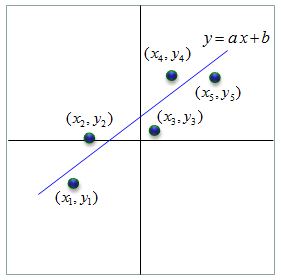Do you have any idea ? The first idea you can think of is just try random values and keep doing 'try and error'. Of course, this is not the one I would recommend. But I suggest you to do random trial at least several times and then you will understand why we need some kind of mathematical approach and will be highly motivated to follow the mathematical procedure all the way to the end even though sometimes it would be hard and sometimes (actually most of times) it become very boring.   There are largely two kinds of methods that are commonly used for this problem. One is 'Calculus' based and the other one is Matrix (linear algebra) based method.     Calculus Method   Basic idea of this method is i) Figure out an equation representing error between the each real data point (data point given to you) and the estimated data point (data point on the estimated line y = a x + b). You will get as many error equation as the given data points. ii) Get each of the error equations squared. iii) Sum up all the squared error equation (at this step, you will have a long/complicated quadratic function with variable 'a' and 'b'. iv) Find the value of 'a' and 'b' that gives you the minimum of the quadratic function.   As a first step, let's look at how 'error' for each data point are defined. The error for each data is defined as shown below. The red point is the estimated point (the ideal data points when we assumed that the regression line is y = a x + b) and the blue point is the data that are given to us.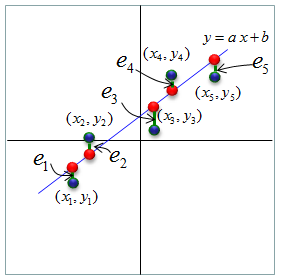Error for each data point is the difference between the red point and blue point. Equation of error for each data point obtained by this method are as follows,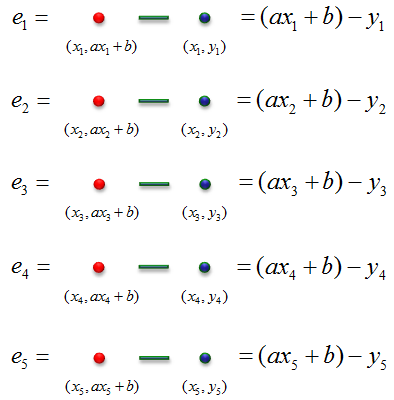Once we have all these error equation, the goal is to find 'a' and 'b' that satisfy the following condition. (As you see, this is to find the least point of sum of squared error. That's how the term 'Least Square' came from).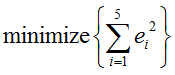At this point, you may ask why we need to get all the error squared. Why not simply adding up all the errors as follows without squaring. This cannot be used because in some case just summing up the errors would give you completely wrong information. For example, let's assume that you have error is 100 and another error = -100. It is pretty big error and the only difference is the direction of the error and only sign of th evalue is different. But if you sum up these two errors as they are, it gives you the result of '0' (e1 + e2 = 100 + (-100) = 0) that may give you the impression that there is no error at all.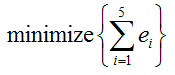Then you may ask why not using following equation. This would resolve the issue that is mentioned above. It is true. It can solve the problem that a negative error is canceled away with a positive error. But this absolute equation is hard to analyze mathematically (especially with Calculus).Now I think we all understand why we need to use 'Squared Sum of Errors' as follows.If you plug in each error equation into the equation above, you would get the following equation. It would look scary, but it can be done by high school math. Just don't get scared and try on your own.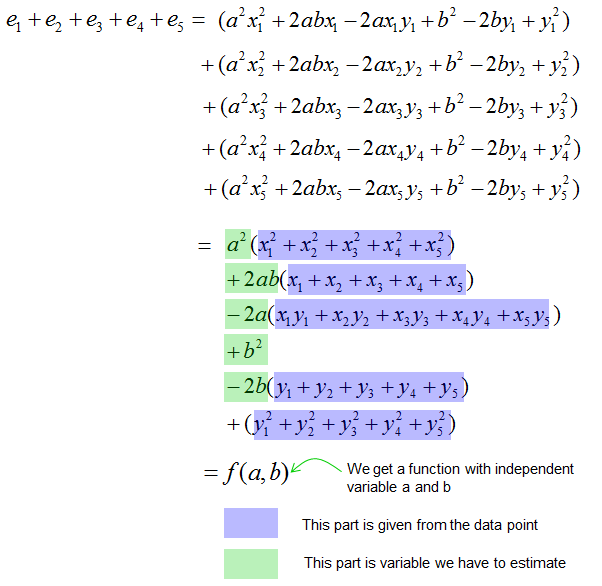If you have a real data value for x(n) and y(n) and plug all those values into the equation shown above. You will have a quadratic equation with two variable 'a' and 'b' and you can plot the quadratic equation as shown below.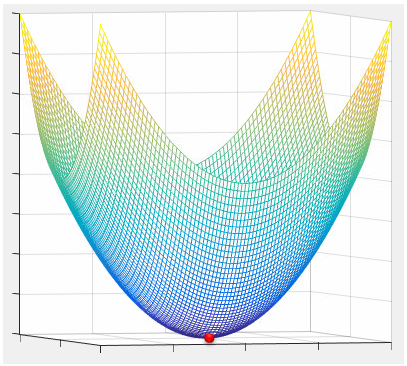Now the problem become 'find the value 'a' and 'b' marked in the red dot shown above and it is the point that is the minimum value point of the quadratic plot'. As you learned from High school math (Pre-Calculus or Calculus), it is the point where it satisfies the following condition. I would not solve this equation any further since the important thing is how you come to this point and meaning of this point. In reality, finding the point would be done by various computer software.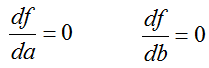Matrix Method   Another method to solve this problem (finding the regression equation) is to use Matrix (Linear Algebra) equation. I would just put down the conclusion of this method and would not go through the detailed 'Proof' and 'Derivation' process. In real situation, this method would be more widely used comparing to 'Calculus' method described above. Also, in most case you would use various kinds of software to find the solution. Most of the real life problem will generate pretty big matrix which is hard to be solved by pen-and-pencil. However, at least you need to know how to construct the matrix equation itself from the given data set even though the calculation process is done by software.   From the given data set and the regression equation that we want to fit the date to (y = a x + b in this example), you can generate equations as shown in (A). And then you can convert the simultaneous equation to a matrix equation as (B). (Refer to Matrix : Simultaneous Equation page if you are not familiar with this conversion).   If you replace the matrix and vector with a character just to make it simple (look less scary), you would get the equation as shown in (C) and (D) which you would see in most of linear algebra textbook.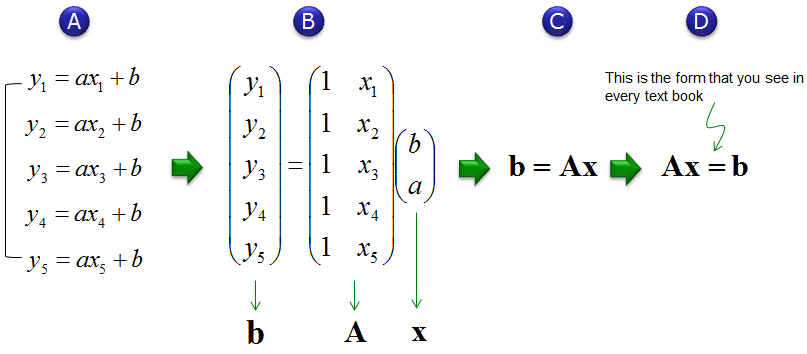Once you get a Matrix Equation as shown above, you can apply the techniques you would learn from most of Linear Algebra textbook.   By muliplying transpose (A) on both side, you can rewrite the equation as below.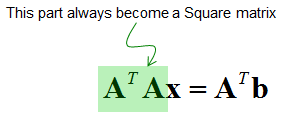Also applying the simple algebric rule, you can find the solution (the vector x) as shown below.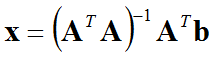You may have a question "I understand how I got the vector x in this process, but I don't understand how we can guarantee the 'x' obtained in this process will be the value that minimized the error (the error between the given data and the corresponding the point on regression line).   Very good question. But I would not go through the details of this. You may find the answer from many textbook or even just by googling.   Example 1 >   Just to give you clearer understanding. I would give you an example. I used Matlab in this example, but I used very small number of data set so that you can even try with pen-and-pencil on your own.   Let's assume that you have following data set which is made up of 5 data points. {(x1,y1), (x1,y1), (x1,y1), (x1,y1), (x1,y1)} = {(-3, -4.2),(-2, -2.4),(-1.0,-2.0),(2.0,1.2),(3, 4.0)} The first step is to generate a matrix equation from this dataset. I strongly suggest you to construct this matrix equation on your own in hand writing. If you get the matrix equation as shown below, you did it right. One very important thing is to keep remind you of the meaning of each elements in each matrix and vectors. Especially the meaning of 'b' and 'a' in the vector 'x'. Otherwise, you would not know how to interpret the result when your software gives you the result of the calculation. In this case, 'b' is the 'y abscissa' of the regression equation and 'a' represents the slope of the regression equation.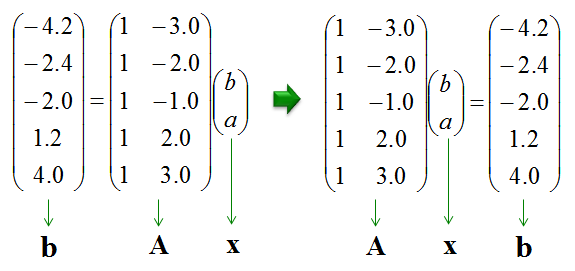Once you get this matrix equation, you can use any software that you like to solve the equation. I used Matlab here and following is the matlab code for this. p = [-3 -4.2;-2 -2.4;-1.0 -2.0;2.0 1.2;3 4.0];   A = [1 p(1,1);1 p(2,1);1 p(3,1);1 p(4,1);1 p(5,1)] b = p(:,2) x = (inv(A' * A) * A') * b % this gives you to solution of the matrix equation.   % This part is not mendatory process, but I just put this to draw a plot to show you how well the solution fits % the given dataset.   t = -5:1:5; y = x(2)*t + x(1);   plot(p(:,1),p(:,2),'bo',t,y,'r-'); axis([-5 5 -5 5]);   Following is the numerical result. Actually A, b is the one I manually set in the code (this is a kind of input) and only the vector 'x' is the calculated value (this is the output)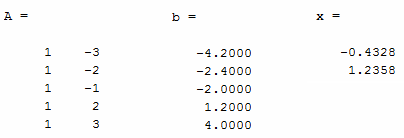Following is the graph showing both  given data set and the regression line. Blue points are the given data and the red line is regression line.